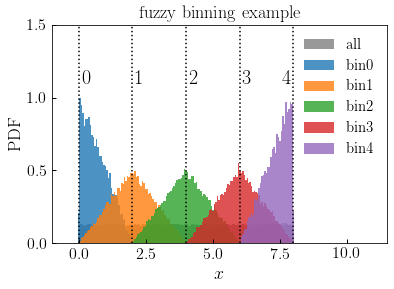# fuzzy_digitize¶

halotools.utils.fuzzy_digitize(x, centroids, min_counts=2, seed=43)[source] [edit on github]

Function assigns each element of the input array x to a centroid number.

Centroid-assignment is probabilistic. When a point in x is halfway between two centroids, it is equally likely to be assigned to the centroid to its left or right; when a point in x is coincident with a centroid, it will be assigned to that centroid with unit probability; assignment probability increases linearly as points approach a centroid.

The fuzzy_digitize function optionally enforces that elements of very sparsely populated bins are remapped to the nearest bin with more than min_counts elements.

Parameters: x : ndarray Numpy array of shape (npts, ) storing the values to be binned centroids : ndarray Numpy array of shape (num_centroids, ). The values of centroids must strictly encompass the range of values spanned by x and must also be monotonically increasing. min_counts : int, optional Minimum required number of elements assigned to each centroid. For those centroids not satisfying this requirement, all their elements will be reassigned to the nearest sufficiently populated centroid. Default is two. seed : int, optional Random number seed. Default is 43. centroid_indices : ndarray Numpy integer array of shape (npts, ) storing the index of the centroid to which elements of x are assigned. All integer values of centroid_indices will lie in the closed interval [0, num_centroids-1].

Examples

>>> npts = int(1e5)
>>> xmin, xmax = 0, 8
>>> x = np.random.uniform(xmin, xmax, npts)
>>> epsilon, nbins = 0.001, 5
>>> xbin_edges = np.linspace(xmin-epsilon, xmax+epsilon, nbins)
>>> centroid_indices = fuzzy_digitize(x, xbin_edges)